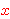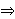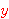# Workshop Miscellany

Norm Megill
nm at alum dot mit dot edu

About this page: This page was originally created as part of a presentation at the 2003 Argonne Workshop on Automated Reasoning and Deduction (AWARD2003), and more material has been added over time. Items 2 through 17 each present one or more open problems (which in some cases have been solved, as noted).
• Updated 11-Apr-2018 - Benoît Jubin solved the problem of item 14, showing the "Axiom of Twoness" is needed for completeness of the ZFC axioms without distinct variables.
• Updated 30-Dec-2007 - Added item 9b.
• Updated 21-May-2007 - Eric Schmidt found two redundant axioms in the list of axioms for complex numbers (see item 11).
• Updated 26-Jul-2006 - Added item 17.
• Updated 21-Jan-2006 - Juha Arpiainen solved the problem of item 9a, proving the independence of axiom ax-11. See also item 9.
• 15-Aug-2005 - My 2005 talks were "Emulating Hilbert's Epsilon in ZFC" (PDF slide show) (LaTeX source file) and "Existential Uniqueness" (PDF slide show) (LaTeX source file).
• Updated 12-Apr-2005 - Raph Levien solved the problem of item 16, answering it in the negative. See also item 9.
• Updated 19-Feb-2005 - N. Megill found a redundant axiom in the list of axioms for complex numbers (see item 11).
• Updated 26-Jun-2004 - expanded the comment under item 13.
• 5-Aug-2004 - My 2004 talk was "Hilbert Lattice Equations" (PDF slide show) (LaTeX source file).
• Updated 6-May-2004 - Kurt Maes found an impressive new version of the Axiom of Choice (see item 8).
• Updated 4-May-2004 - Greg Bush has found shorter proofs for 67 of the 193 propositional calculus theorems in Principia Mathematica (item 4). See this note.
• As of 31-Jan-2004, all problems on this page are still open.
• Updated 1-Jan-2004 - added item 16.
• Updated 10-Aug-2003 - added item 9a.
• Updated 18-Jul-2003 - added item 15 (regarding the $100 prize problem). This page was part of an informal talk I gave at the AWARD-2003 Workshop on Jul. 12, 2003. My other (main) talk was "Orthomodular Lattices and Beyond" (PDF slide show) given on Jul. 11. (To make the slide show, I used the command "pdflatex megillaward2003.tex".) Back to the AWARD-2003 Workshop page. And the AWARD-2004 Workshop page. And the AWARD-2005 Workshop page. These are a few miscellaneous things you can explore at your leisure. 1. (deleted) 2. Open problem: Does a single axiom (e.g. Sheffer-stroke) exist for weakly orthomodular lattices (WOML)? What I mean is a single identity added to the usual axioms and rules of inference for identity (reflexivity, symmetry, transitivity, substitution). In other words, it would be a single identity which, together with these axioms, would determine the equational variety WOML of weakly orthomodular lattices. It would be exactly analogous to McCune's Single Axiom for Orthomodular Lattices with "WOML" substituted for "OML". It was this page, in fact, that led me to wonder about an analogous equation for WOML. 3. Kalmbach's CN (implication, negation) quantum propositional calculus with modus ponens as sole rule of inference. Open problems: (1) independence of axioms; (2) more elegant, shorter axiomatization; (3) is A16 needed for completeness? (4) is the system D-complete? (5) is there a single axiom!? Another note on Kalmbach's system is here. 4. Using the standard three classical propositional calculus axioms, here are condensed detachment proofs of the 193 propositional calculus theorems in Principia Mathematica. Open problem: Are these the shortest proofs? Update 4-May-2004: Greg Bush has found shorter proofs for 67 of these. See this note. 5. The 3-variable (weak) orthoarguesian law ax-3oa can be derived from the modular law av(b^(avc))=(avb)^(avc) added to an OL. Open problem: can the standard orthoarguesian law ax-4oa be derived from the modular law + OL? 6. The Arguesian law is the same as the orthoarguesian law in the form of oa6 with its 3 hypotheses removed. It has been proved to be strictly stronger than the modular law, and the modular law can be derived from it. Open(?) problem: does there exist a finite ortholattice that is modular but non-Arguesian? [This lattice might also answer the previous question (5)]. 7. Open problem: can Godowski's 3-variable equation (see slide 17 in my AWARD-2003 Workshop presentation (PDF)) be derived from OL + modular law? How about the 4-, 5-,... variable versions? How about the new equation (32) in slide 17? 8. A short equivalent of the Axiom of Choice. Open problem: Does a shorter equivalent (expressed in first-order logic primitives) exist? (Here's a another cute version using some abbreviations.) Update 6-May-2004: In April 2004, Kurt Maes found a version of the Axiom of Choice that has about the same length. More importantly, his version has only 5 quantifiers in prenex normal form, setting a new record. See this theorem ackm. 9. Metamath's axiom system. If the apparent willy-nilly mixing of free and bound variables confuses you, read this. Open problems: (1) Are the axioms for predicate calculus independent? (2) Can the axiomatization be simplified? Update 12-Apr-2005: Raph Levien proved that ax-9 is independent from the others; see item 16 below. Now we only have 12 more (out of 13) independence proofs to go! Update 21-Jan-2006: Juha Arpiainen proved that ax-11 is independent from the others; see item 9a below. Now we only have 11 more (out of 13) independence proofs to go! 9a. The open problem stated in Remark 9.5 on p. 16 (PDF p. 17) of "A Finitely Axiomatized Formalization of Predicate Calculus with Equality" is still open (as of 10-Aug-2003). In terms of Metamath's axiom system, the problem asks whether ax-11 can be proved from ax-1 through ax-10, ax-12 through ax-15, ax-mp, and ax-gen, and no others. It should be noted ax-11 is not logically independent, because any specific instance of ax-11, where phi is replaced with a subformula containing only set (meta)variables but no wff (meta)variables, is provable from the others mentioned. Update 21-Jan-2006: Juha Arpiainen solved this problem and proved that ax-11 is metalogically independent from the others. Hence, there cannot exist a proof of ax-11 from the others. Here is his seven-line proof:   Ax-11 need not hold if there are additional predicates that do not satisfy the analogues of ax-13 and ax-14. For example, consider the system with one additional binary predicate "x ~ y". The set of ordered pairs of integers is then a model of ax-1 to ax-10, ax-12 to ax-17 if "x = y" and "x e. y" are interpreted as "the first components of x and y are equal" and "x ~ y" as "the second components are equal". However, equs5 (and therefore also ax-11) is false for the wff "x ~ y". 9b. An open problem is whether ax-11o can be derived in a predicate calculus system that omits ax-16 and ax-17 (but includes ax-11). See the 5th table entry in Appendix 7: Some Predicate Calculus Subsystems 10. A weak deduction theorem for classical propositional calculus. Example of its use. Open problem: In quantum propositional calculus, the standard deduction theorem fails (and so does this weak deduction theorem). What kind of "weak" deduction theorems are possible? 11. Axiomatization for complex numbers. Open problem: are the axioms independent? (N. Megill reduced the number from 28 to 27 on 19-Feb-2005. Eric Schmidt weakened "1 is real" to "1 is complex" on 11-Apr-2007. Eric Schmidt reduced the number of axioms from 27 to 25 on 21-May-2007.) 12. Consider the "pure" fragment of Metamath axiom system, ax-1 through ax-7 (and ax-mp, ax-gen). Open problem: Can this fragment directly prove all such theorem schemes of its kind*, i.e. is it complete for this fragment? (I think so.) [* No predicate connectives (such as =), no distinct variable restrictions.] Possible clue (suggested by Bob Meyer): this fragment seems analogous to modal logic S5 (or monadic predicate calculus), extended by introducing the second variable y in ax-7. 13. Set theory can be added to standard first order logic without equality by making the membership epsilon the only primitive predicate and introducing equality as a defined symbol. The usual axioms of equality then become theorems of set theory (see comments under the Axiom of Extensionality). This provides a system that is quite beautiful from a minimalist point of view. However, in Metamath's axiom system predicate calculus there is no primitive notion of proper substitution, and additional equality axioms serve this role (see the definition of proper substitution and related theorems). So dispensing with equality would almost certainly require some additional axioms with epsilon, beyond Extensionality, to fill the gap. Problem: What would a "nice" set of epsilon-only axioms look like? (The existing axioms could be expanded with the definition of equality, of course, but they would be long and ugly with possible redundancies.) Philosophical problem: Would these additional axioms be part of set theory or logic? If they are considered part of logic, in what ways does such a "minimal" predicate calculus, with a single arbitrary binary predicate calculus, differ from standard predicate calculus? How much of proper substitution is "pushed" into set theory, and what is the character of the non-set-theory part left over? 14. In this odd formalization of set theory axioms with no distinct variable restrictions, is the "Axiom of Twoness" essential for completeness? (I think so.) Update 11-Apr-2018: Benoît Jubin proved this is the case. His proof is as follows:   In a model with a single element which does not contain itself, all axioms and rules of predicate calculus, all axioms of "ZCF-no$d" except twoness, and all axioms of standard ZFC except ax-pow and ax-inf are satisifed. In other words, strip out all quantifiers, evaluate all "X = Y" to True and all "X \in Y" to False. Then, all axioms evaluate to True except ax-pow and ax-inf. Interestingly, the model with a single element containing itself satisfies all axioms except, of course, ax-reg (and ax-reg-no$d). What is interesting is that it satisfies ax-inf (and ax-inf-no$d), but of course it does not satisfy the real infinity axiom ax-inf2. This proves that ax-reg is necessary in the derivation of ax-inf2.

15. Stuff related to the OI3 conjecture (slide 22 of PDF file Orthomodular Lattices and Beyond) [whose solution will earn a $100 prize (slide 23)]: 1. As a "sanity check" it might be useful to show Otter can prove OI3 from OA3+OML. The proof is relatively simple (for Otter too, I think) and is shown in Th. 4.10, p. 26, of http://xxx.lanl.gov/abs/quant-ph/0009038. 2. It might be interesting to see which of the known implications on slide 26 of http://www-unix.mcs.anl.gov/~mccune/award-2003/megill.pdf that Otter is able to do. Some are easy, but others seemed tricky (to do by hand anyway). I can provide the proofs of any of them if desired. 3. I would be interested in seeing a proof of (or counterexample to) any of the open implication directions on slide 26, such as 48+OML => 47 or 45+OML => 49. (If a counterexample is found, that would of course also disprove the OI3 conjecture and be eligible for the prize.) 4. I would also be interested in any combinations of equations in slide 26 that together might produce others, in directions that are currently unknown. For example, can OML+46+49+53 prove 44? 5. The equations in slide 26 that aren't known OA3 equivalents are 45, 46, 47, 48, 49, 50, 51, and 53. It would be interesting to see if any equations in this set, or even all of them together, could (when added to OML) prove the equation of slide 24. (The equation of slide 24 can be derived from OA3+OML.) It would not solve the OI3 conjecture but might provide further clues. 16. (Predicate calculus) In A Note on the (Metamath) Axioms, 3rd paragraph, an open problem is whether "x4 x4 = x4" can be proved from "x3 x3 = x3" in the absence of ax-9. Or expressed as a Metamath problem: canbe proved without using ax-9? This can be expressed equivalently as the following problem in the Metamath language:  ${ exeqid.1 $e |- E. x x = x$. $( Open problem 16 in award2003.html$) exeqid $p |- E. y y = y$= ? $.$}

Update 12-Apr-2005: Raph Levien proved this is impossible. His proof is as follows:

  Assume that we have a proof of E. y y = y starting from the hypothesis E. x x = x. Expand out the proof so that all proof steps are invocations of axioms 1-8, 10-12, 16-17, mp and gen, and all metavariables are replaced by actual variables, including x_4 as the replacement for y. Now transform all proof steps so that any equality involving x_4 becomes "false", and check whether the resulting proof still holds. All of the axioms not involving equality should be ok. ax-8 is ok because in all cases where the consequent becomes false, at least one of the antecedents also becomes false. ax-10 is ok because if either x or y is x_4, then the antecedent is false. ax-11 is ok because the second antecedent is false. ax-12 is trickier, but goes like this: if z is distinct from x and y, then the consequent follows from ax-17 even if z is x_4. If x or y is x_4, then the third antecedent is false. Lastly, ax-16 is ok because if x or y is x_4, then A. x x = y becomes false. Thus, all transformed proof steps are true when interpreted in standard axioms. However, the transformed E. x_4 x_4 = x_4 is false, which is a contradiction.

If you find the "meaning" of x_4 as a bound variable in Raph's proof is troublesome, notice that all of the axioms and rules of logic (other than ax-9 itself) hold if you simply strip out the quantifiers. So with quantifiers stripped out, a purported proof would prove x_4 = x_4 from x_3 = x_3. Then, using a model where x_4 does not equal anything, Raph's contradiction follows.

This also shows (as obvious corollaries) that ax-9 is independent from the others, and also that we need ax-9 to prove x = x.

17. (Predicate calculus with equality) In Appendix 3: Traditional Textbook Axioms of Predicate Calculus with Equality, last paragraph, an open problem is whether any or all of axioms ax-4 through ax-17 can be proved (directly in Metamath) from the "traditional axioms" expressed as theorems stdpc4, stdpc5, ax-gen, stdpc6, and stdpc7, using dfsb7 as the definition for proper substitution. (The reason to choose dfsb7 over df-sb is to be as conventional as possible.) This is interesting because the traditional axioms provide a logically complete system of predicate calculus that is familiar to more people. We could separate the axioms for predicate calculus and equality into two groups, the traditional logically complete set and a supplemental "technical" set consisting of that subset of ax-4 through ax-17 (or some variant of them) needed to achieve metalogical completeness.